# Spherical Trigonometry

Also found in: Dictionary, Thesaurus, Acronyms, Wikipedia.
Related to Spherical Trigonometry: spherical geometry, spherical triangle

## spherical trigonometry

[′sfir·ə·kəl ‚trig·ə′näm·ə·trē]
(mathematics)
The study of spherical triangles from the viewpoint of angle, length, and area.

## Spherical Trigonometry

the mathematical discipline that studies the relationships between angles and sides of spherical triangles.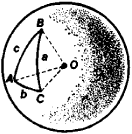Figure 1

Suppose A, B, and C are the angles of the spherical triangle /tBC(see Figure 1), and suppose a, b, and c, respectively, are the sides opposite these angles. The following relations hold between the angles and sides of the triangle: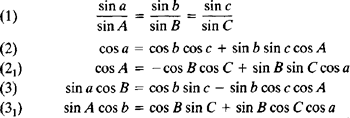In these fundamental formulas, the sides a, b, and c are measured by the corresponding angles subtended at the center of the sphere. The lengths of these sides are aR, bR, and cR, respectively, where R is the radius of the sphere. If the designations of the angles and of the sides are changed through cyclic permutations (that is, A→B→+C→A and a→b→c→a), other formulas of spherical trigonometry, which are similar to the above, can be given. If any three elements of a spherical triangle are known, the formulas of spherical trigonometry permit the other three elements to be found—that is, the formulas make it possible to solve the triangle.

The formulas of spherical trigonometry are simplified in the case of right spherical triangles. When A = 90° and a is the hypotenuse, we have, for example,

(1’) sin b = sin a sin B

(2’) cos a = cos b cos c

(3’) sin a cos B = cos b sin c

The formulas needed to solve a right spherical triangle can be obtained from Napier’s rules. A description of one of the rules follows. Omitting the right angle, we imagine the five “parts”—that is, the other two angles, the hypotenuse, and the complements of the other two sides—as being arranged on a circle in the same order as on the triangle: B, a, C, 90° – b, 90° – c. Napier’s rule states that the cosine of each part is equal to the product of the sines of the nonadjacent parts, for example,

cos a = sin (90° – c) sin (90° – b)

It follows that

cos a = cos b cos c

which is formula 2’.

Gauss’ formulas, also known as Delambre’s analogies, state relations between all six elements of a spherical triangle and are useful in solving problems. The formulas are as follows: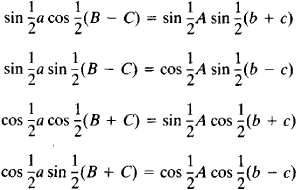In solving many problems in spherical trigonometry, it is often possible, depending on the degree of accuracy required, to use approximate formulas. For small spherical triangles, that is, triangles whose sides are small compared to the radius of the sphere, the formulas of plane trigonometry may be used. In the case of narrow spherical triangles, that is, triangles one of whose sides, say a, is small compared to the other two, the formulas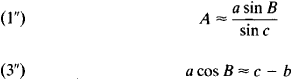or the more accurate formulas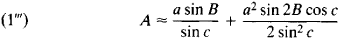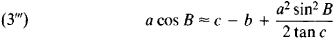may be used.

Spherical trigonometry developed before plane trigonometry. The first-century Greek mathematician Menelaus and the second-century Greek mathematician Ptolemy were familiar with the properties of right spherical triangles expressed by formulas (l’)–(3’) and with various cases of the solution of spherical triangles. The Greek mathematicians reduced the solution of oblique spherical triangles to that of right spherical triangles.

The Azerbaijani mathematician Nasir al-Din al-Tusi of the 13th century systematically studied all cases of the solution of oblique spherical triangles. He was the first to give the solution for the two most difficult cases. The fundamental formulas for oblique spherical triangles were found through the efforts of several mathematicians. The Arab Abu al-Wafa obtained formula (1) in the tenth century. Formulas of type (2) were given by the German Regiomontanus in the mid-15th century. The Frenchman F. Vieta gave formulas of type (21) in the second half of the 16th century. L. Euler presented formulas of type (3) and (31) in Russia in the 18th century.

In 1753 and 1779, Euler gave a complete system of formulas of spherical trigonometry. Some formulas useful in practical applications were established by the Scottish mathematician J. Napier in the late 16th and early 17th centuries, by the English mathematician H. Briggs in the same period, by the Russian astronomer A. J. Lexell in the second half of the 18th century, and by the French astronomer J. Delambre in the late 18th and early 19th centuries.

References in periodicals archive ?
Arcturus is fettered at 10 |degrees~ altitude at each observer latitude and the corresponding altitude of the other star calculated from modern tables or directly by spherical trigonometry. Any value of the altitude of the fettered star could have been chosen, including the one which at the initial point has the same altitude as that of the unfettered star, Canopus.
His topics include characteristics of geographic information, the geometry of common shapes, plane and spherical trigonometry, differential and integral calculus, curves and surfaces, map projections, and correlation and regression.
In response to reader requests, this is the first of a two part series on spherical trigonometry (trig), an important tool in understanding electronic-warfare (EW) engagements and the operation of some types of EW equipment.

Site: Follow: Share:
Open / Close